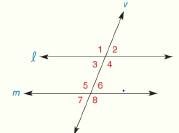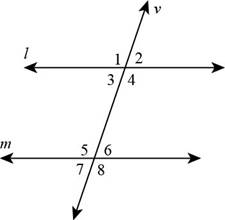Chapter 2.CT, Problem 1CT### Elementary Geometry for College St...

6th Edition
Daniel C. Alexander + 1 other
ISBN: 9781285195698

#### Solutions

Chapter
Section### Elementary Geometry for College St...

6th Edition
Daniel C. Alexander + 1 other
ISBN: 9781285195698
Textbook Problem
3 views

# Consider the figure shown at the right.a) Name the angle that corresponds to ∠ 1 .b) Name the alternate interior for ∠ 6 .To determine

a)

To find:

The angle corresponds to 1 in the given figure.

Solution:

The 5 is corresponds to 1.

Explanation

Given:

The following figure shows the given diagram.Calculation:

The angles that lie in the same relative positions are called corresponding angles for the given lines. Therefore, 5 and 1 lie in the same relative positions about the transversal. Therefore, 5 corresponds to 1.

Conclusion:

Hence, 5 corresponds to 1.

To determine

b)

To find:

The alternate interior angle for 6.

Solution:

The alternate interior angle for 6 is 3.

Explanation

Given:

The following figure shows the given diagram.Calculation:

Alternate interior angles are that angles which have different vertices and lie on the opposite side of the traverse. 6 and 3 have different vertices and lie on the opposite side of the transverse. Therefore, the alternate interior angle for 6 is 3.

Conclusion:

Hence, the alternate interior angle for 6 is 3.

#### The Solution to Your Study Problems

Bartleby provides explanations to thousands of textbook problems written by our experts, many with advanced degrees!

Get Started

#### Evaluate the expression sin Exercises 116. (23)2

Finite Mathematics and Applied Calculus (MindTap Course List)

#### If g(x)=f(x), where the graph off is shown, evaluate g(3).

Single Variable Calculus: Early Transcendentals, Volume I

#### True or False:

Study Guide for Stewart's Single Variable Calculus: Early Transcendentals, 8th

#### True or False: is a geometric series.

Study Guide for Stewart's Multivariable Calculus, 8th